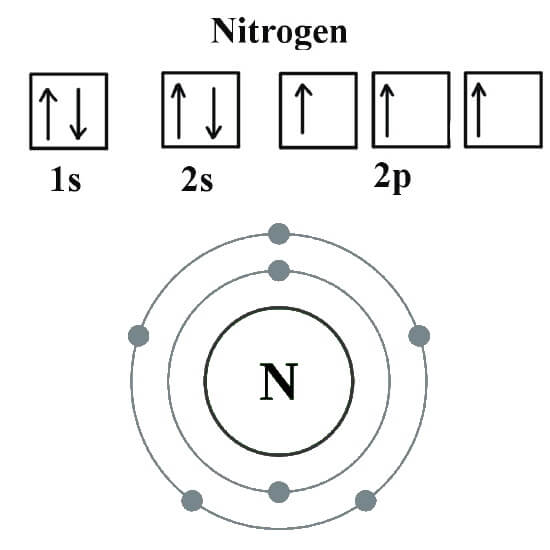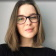# How many valence electrons does nitrogen have?

What is the valency of Nitrogen and how to find the valence electrons for Nitrogen?

﻿
1. Valency of Nitrogen (N) is 3 as it has 5 electrons in its valence shell. Therefore, it can accept 3 electrons to complete its octet.

There are 2 ways to find the number of valence electrons in Nitrogen.

1. Use the Mendeleev’s Periodic Table to figure out how many electrons Nitrogen (N) has in its valence shell. To do so, look at the column number. Nitrogen is in column 15 (also called Group 5A) and therefore Nitrogen has 5 valence electrons.

2. You can also look at the electron configuration of Nitrogen to determine the number of valence electrons. The number of electrons in the highest energy level gives you the number of valence electrons. Using this method you will also determine that Nitrogen has 5 valence electrons.

The full electron configuration for Nitrogen is 1s22s22p3.

1. 1s22s22p3 is the ground-state electron configuration for Nitrogen. As you can see in the image given below.A Nitrogen atom has five valence electrons. To figure that out you should to count across the row that atom is in and stop at it and the number you counted up to is the number of valence electrons. So… Lithium is the first, Beryllium is the second, Boron is the third, Carbon is the fourth and Nitrogen is located in the fifth tall column.

Psst... Stuck with your assignment? 😱Erica
OnlinePsst... Stuck with your assignment? 😱
Do you need an essay to be done?
Yes
Other assignmentWhat type of assignment 📝 do you need?
• Article review
• Case study
• Coursework
• Report
• Research Paper
• Other AssignmentHow many pages (words) do you need? Let's see if we can help you!